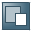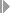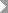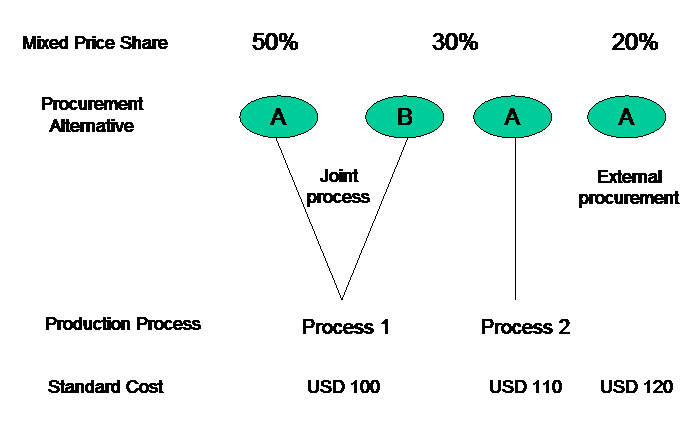Show TOC

###Variance CategoriesThe system assigns each variance to a variance category. The variance category indicates the cause of the variance (such as price change or changed lot size). Variances are updated broken down into variance categories.

#### Prerequisites

You have made the following settings in Customizing for Product Cost By Period or Product Cost by Order:

• Product Cost by Period:

• You have selected the variance categories you want to calculate underPeriod-End ClosingVariance CalculationVariance Calculation for Product Cost CollectorsDefine Variance VariantsorVariance Calculation for Cost Object HierarchiesDefine Variance Variants.

• You have defined variance keys underPeriod-End ClosingVariance CalculationVariance Calculation for Product Cost CollectorsDefine Variance KeysorVariance Calculation for Cost Object HierarchiesDefine Variance Keys.

• Product Cost by Order:

• You have selected the variance categories you want to calculate underPeriod-End ClosingVariance CalculationCheck Variance Variants.

• You have defined variance keys underPeriod-End ClosingVariance CalculationDefine Variance Keys.

#### Integration

The variances calculated with target cost version 0 can be transferred to Profitability Analysis broken down into variance categories.

#### Features

Variances are divided into variance categories on the input side and variance categories on the output side.

##### Variances on the Input Side

Variances on the input side are variances based on goods issues, internal activity allocations, overhead allocation, and G/L account postings. These variances are assigned to the following variance categories according to their cause:

• Input price variances

Input price variances are caused by differences between the planned and actual prices of the materials and activities used.

The system can only calculate input price variances if quantities were specified in addition to the planned and actual costs. The following conditions must be met for this:

• Select the Material origin indicator in the costing view of the master record of all materials that have a large influence on your costs.

Uniform units of measure must be assigned to all input materials. That is, material costs can only be totaled under a cost element when such costs are carried under the same unit of measure.

• If you want to calculate variances by cost element/origin group, you must already have entered an origin group in the costing view of the material master record for all material components.

If you have defined primary input price variances in Customizing, the system will calculate input price variance percentages and update them with reference to the operation. When you start the variance calculation process at the end of the period, this data will be canceled and the input price variances calculated by multiplying the difference between the planned price and the actual price by the confirmed quantity. If the confirmed quantities are incomplete or do not exist, input price variance percentages will be shown.

• Resource-usage variances

Resource-usage variances are caused by the use of different materials and activities than were planned.

The system calculates resource-usage variances if:

• The target costs of a cost element, origin group, or origin are not zero while the actual costs of the same cost element, origin group, or origin are zero.

• The target costs of a cost element, origin group, or origin are zero but the actual costs of the same cost element, origin group, or origin are not zero.

• Input quantity variances

• Input quantity variances are caused by differences between the planned and actual consumption quantities of materials and activities. Select the Material origin indicator in the costing view of the master record of all materials that have a large influence on your costs.

If you want to see input quantity variances and input price variances, you must make the following settings:

• Select the Material origin indicator in the costing view of the material master record.

• Select the Record quantity indicator in the cost element.Note

When it calculates the variances, the system does not check whether the Record quantity indicator is selected. If quantities are not recorded, however, it is not possible to calculate quantity variances. If the Record quantity indicator is selected, a warning message is issued if you do not enter a quantity in consumption postings.

If the above requirements are not met, the variances are reported as remaining input variances.

End of the note.
• Remaining input variances

The system calculates variances for each cost element on the input side. If the system cannot assign a variance on the input side to any of the above categories, it assigns the variance to the remaining input variance category. This can happen when costs are entered without a quantity, or when the overhead rates are changed.

• Scrap variances

Scrap variances are caused by differences between the operation scrap planned in the routing and the operation scrap actually confirmed.

The system reports the difference between the planned scrap quantity and the actual scrap quantity as an unplanned scrap quantity. The unplanned scrap quantity is valuated in accordance with the Valuation variant for work in process and scrap in Customizing for Product Cost by Period or Product Cost by Order underPeriod-End ClosingVariance Calculation.

If you have not activated the calculation or display of scrap, scrap variances are assigned to the individual variance categories on the input side.

For detailed information on scrap variances, refer to the section Scrap Variance.

##### Variances on the Output Side

With the following types of variances the system will show variances on the output side:

• Total variance (SAP standard: target cost version 0)

• Production variance of period (SAP standard: target cost version 3)

• Planning variance (SAP standard: target cost version 2)

Variances on the output side are variances that result from too little or too much of the planned order quantity being delivered, or because the delivered quantity was valuated differently.

• Mixed-price variances

Mixed-price variances arise when the system valuates inventories using a mixed cost estimate for the material. A mixed-price variance is caused by a difference between the target credit (Confirmed Quantity × Standard Cost of Procurement Alternative) determined by variance calculation, and the actual credit that was posted when the goods were received (Confirmed Quantity × Standard Price). The standard price corresponds to the mixed price.

If you have not selected the Mixed-price variance field in the variance variant, mixed-price variances are reported as output price variances.

• Output price variances

Output price variances are reported in the following situations:

• If the standard price has changed between the time point of delivery to stock and the time point when the variances are calculated

• If the material’s price control indicator is set to V and the material was not delivered to stock at the standard price

• If the price used to valuate the inventory is not a mixed price

Output price variances are caused by a difference between the target credit (such as Confirmed Quantity x Standard Price) determined by variance calculation, and the actual credit posted when the goods were received (such as Confirmed Quantity x Moving Price).

You control which value is used for delivery to stock when the price control indicator is set to V in Customizing for Product Cost by Period or Product Cost by Order under Define Valuation of Goods Received for Order Delivery.

• Lot size variances

Lot size variances are differences between the planned costs that do not vary with the lot size and the actual costs that do not vary with the lot size, which are passed on to inventory when you put the order quantity into inventory. A lot size variance arises when a portion of a material’s total cost does not change when the you change the quantity of goods manufactured.

Lot size variances are calculated in the following formula:

Lot Size Variance = Lot-Size-Independent Target Costs × (1 - Control Quantity ÷ Planned Quantity)

Lot size variances are only calculated if the planned quantity is different than the confirmed quantity (the delivered quantity).

• Remaining variances

Differences between the target costs and the allocated actual costs that cannot be assigned to any other variance category. For example, rounding differences are reported as remaining variances.

Overhead applied to costs that do not vary with the lot size are also reported as remaining variances.

Remaining variances are reported in the following situations:

• When the system cannot calculate target costs, such as when no cost estimate for the material exists or because no goods receipt for the order has taken place in the Product Cost by Period component.

• When no variance categories have been defined in the variance variant in Customizing for Cost Object Controlling.

If you do not select a variance category, the variances of that category are assigned to the category remaining variances. The scrap variances are an exception to this. Scrap variances that are not shown as such can flow into any other variance category on the input side.

#### Example

##### Example for Variance Categories on the Input Side
• Input price variance

Raw material 1 goes into the standard cost estimate at USD 10 (the standard price is selected according to the valuation strategy). For the goods issue, the price control indicator specifies that the goods movement is valuated with the moving average price. The moving average price for raw material 1 is USD 11 at the time of withdrawal. The system reports an input price variance of USD 1.

• Resource-usage variance

Example 1:

Raw material 1 was not available, so raw material 2 was used instead. The first raw material is assigned to cost element 400000 and the second raw material to cost element 412000. The system reports the costs for raw material 1 and raw material 2 as resource-usage variances. Taking the order as a whole, the two amounts together result in a resource-usage variance.

Example 2:

You are using separate valuation. The valuation type in the actuals specifies a different account than the totals record that was used for the standard cost estimate. Because different accounts and therefore different cost elements were used, the system reports a resource-usage variance.

• Input quantity variance

Machine time of 15 minutes was planned. 17 minutes were confirmed. The machine time costs USD 5 per minute. This results in an input quantity variance of USD 10.

• Remaining input variance

Example:

The material overhead is higher than planned because the material price for raw material 1 was changed. The system reports the difference between the planned material overhead and the actual material overhead as a remaining input variance.

• Scrap variance

See: Scrap Variances

##### Example for the Assignment of Costs on the Input Side

Suppose you have planned a cost of USD 10 per piece for raw material 1. The actual cost for raw material 1 is USD 11. The planned consumption for raw material 1 was 10 pieces, but the actual consumption is 11 pieces. The planned cost is USD 100. The actual cost is USD 121. In both cases the order quantity is 10 pieces. The difference contains both an input price variance and an input quantity variance.

The input price variance is USD 10, the input quantity variance is USD 10, and USD 1 could be considered either an input price variance or an input quantity variance.

Because the system calculates the input price variances first, it assigns the USD 1 to the input price variance category, resulting in an input price variance of USD 11 and an input quantity variance of USD 10.

##### Examples for Variance Categories on the Output Side
• Mixed-price variance

Material A has multiple procurement alternatives. A mixing ratio defines the relationship between the procurement alternatives. A mixed cost estimate is created on the basis of the mixing ratio. The mixed cost estimate calculates a mixed price. The mixed price corresponds to the standard price of the material.The mixed price is calculated as follows:

USD 100 × 5 = USD 500

USD 110 × 3 = USD 330

USD 120 × 2 = USD 240

USD 500 + 330 + 240 = USD 1,070

USD 1,070 ÷ 10 = USD 107

The mixed price is USD 107.

You create a production order for the procurement alternative that is included in the mixing ratio at 30%.

Production order 4711 for material A

Planned quantity

10 units

Actual quantity

10 units

Actual cost (debit)

USD 1,150

Credit from goods receipt

USD 1,070

Balance

USD 80

The variance calculation process goes through the following steps:

The total variance is the difference between the debit and credit. This amount is USD 80. You want to have this total variance explained with variance calculation (target cost version 0). In variance calculation the target costs are compared with the control costs.

Target costs = Confirmed quantity (yield) x Standard cost of procurement alternative

Result:

Target cost = 10 × USD 110 = USD 1,100

Production Process

If you compare the target costs on the order with the debited actual costs, the result is a variance of USD 50. This difference corresponds to the variances that are not mixed-price variances.

If you compare the target costs with the allocated actual costs, the result is a variance of USD 30. This difference between the target costs and the credit of the order is the mixed-price variance. This variance arises because the standard cost of the procurement alternative for which an order was created is not the standard price.

 Posted actual cost (debit) USD 1,150 Allocated actual cost (goods receipt) USD 1,070 Target cost USD 1,100 Control cost USD 1,150 Variances on the input side USD 50 Mixed-price variance USD 30

The sum of the variances on the input side and the mixed-price variance explain the balance on the order that results from the difference between the debit and the credit.

• Output price variances

In a standard cost estimate for a material, you have calculated a standard price of USD 75. The system delivers 10 units to stock for production order 4711, and valuates this delivery with the standard price. You calculate a new standard cost estimate for the material, mark the cost estimate, and release it. The new standard price is USD 100. The system calculates a target cost of USD 1,000. The output price variance is USD 250.

• Lot size variances

The setup time and teardown time for an operation remain the same, regardless of how many times an operation is carried out.

For example, if the order quantity is less than the costing lot size, the setup costs and the teardown costs based on one unit of the product for the order will be higher than in the cost estimate. The system reports this difference as a lot size variance.

For detailed information on the variance categories for scrap, see the Implementation Guide for the Product Cost by Period component and the Implementation Guide for the Product Cost by Order component underPeriod-End ClosingVariancesVariance Variant.

For detailed information on the valuation variant for scrap, see the Implementation Guide for the Product Cost by Period component and the Implementation Guide for the Product Cost by Order component underPeriod-End ClosingVariancesDefine Valuation Variant for Scrap (Target Costs).

For information on the special features of variance calculation and scrap calculation with a valuated sales order stock, refer to the following section:

Variances with a Valuated Sales Order Stock

For information on valuation and standard price calculation with a valuated sales order stock, refer to the following sections:

Update of Valuated Sales Order Stock

Standard Price with Valuated Sales Order Stocks